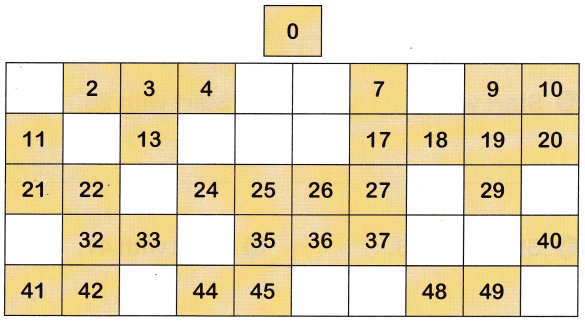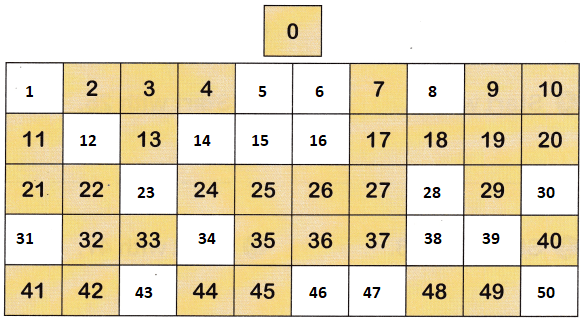All the solutions provided in McGraw Hill Math Grade 1 Answer Key PDF Chapter 4 Test as per the latest syllabus guidelines.

Tell what number comes next. Write the number.

Question 1.
0 ____
The number next to 0 is 1

Question 2.
5 ____
The number next to 5 is 6.

Question 3.
9 ____
The number next to 10 is 11.

Question 4.
3 ___
The number next to 3 is 4.

Question 5.
15 ___
The number next to 15 is 16.

Question 6.
18 ___
The number next to 18 is 19.

Question 7.
7 ____
The number next to 7 is 8.

Question 8.
11 ___
The number next to 11 is 12.

Question 9.
Write the missing numbers.The list of the missing numbers are shown in the below table,Count. Write the numbers.

Question 10.
0 1 ___ __ ___ ___
Forward counting is counting by adding one more, every time.
The numbers next to 0 1 is 2 3 4 5

Question 11.
13 14 ___ ___ ___ ___
Forward counting is counting by adding one more, every time.
The numbers next to 13 14 is 15 16 17 18

Question 12.
34 35 ___ ___ ___ ___
Forward counting is counting by adding one more, every time.
The numbers next to 34 35 is 36 37 38 39

Question 13.
27 28 ___ ___ ___ ___
Forward counting is counting by adding one more, every time.
The numbers next to 27 28 is 29 30 31 32

Question 14.
39 40 ___ ___ ___ ___
Forward counting is counting by adding one more, every time.
The numbers next to 39 40 is 41 42 43 44.

Question 15.
18 19 ___ ___ ___ ___
Forward counting is counting by adding one more, every time.
The numbers next to 18 19 is 20 21 22 23

Question 16.
6 7 ___ ___ ___ ___
Forward counting is counting by adding one more, every time.
The numbers next to 6 7 is 8 9 10 11

Question 17.
45 46 ___ ___ ___ ___
Forward counting is counting by adding one more, every time.
The numbers next to 45 46 is 47 48 49 50.

Question 18.
___ ___ ___ ____
Forward counting is counting by adding one more, every time.
The number starting with 7 is 7 8 9 10

Question 19.
___ ___ ___ ____
Forward counting is counting by adding one more, every time.
The number starting with 19 is 19 20 21 22

Question 20.
___ ___ ___ ____
Forward counting is counting by adding one more, every time.
The number starting with 30 31 32 33

Question 21.
___ ___ ___ ____
Forward counting is counting by adding one more, every time.
The number starting with 21 is 21 22 23 24

Question 22.
___ ___ ___ ____
Forward counting is counting by adding one more, every time.
The number starting with 42 is 42 43 44 45

Question 23.
___ ___ ___ ____
Forward counting is counting by adding one more, every time.
The number starting with 16 is 16 17 18 19

Question 24.